Saturday 28th November 2020
 CBSE Guess > Papers > Question Papers > Class XII > 2003 > Physics > Delhi Set -I PHYSICS—2003 (Set I—Delhi)
Q. 1. Define electrical conductivity of a conductor and give its S.I. unit. Q. 2. What happens to the power dissipation if the value of electric current passing through a conductor of constant resistance is doubled? Q. 3. How does the (i) pole strength and (ii) magnetic moment of each part of a bar magnet change if it is cut into two equal pieces transverse to its length? Q. 4. Write S.I. unit of magnetic flux. Is it a scalar or a vector quantity? Q. 5. Name the factor which decides the quality of reproduced document sent by Fax. Q. 6. Mention any two properties of electric lines of force. Sketch them for an isolated positive point charge. Q. 7. In a copper voltameter, a varying electric current, as shown in graph, is passed. The mass of copper deposited at the end of 30 seconds is m grams. Using the graph, find the value of e.c.e. of copper in gC -1 .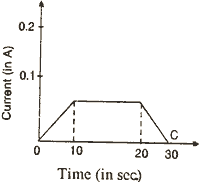O. 8. An a.c. voltage E = E0 sin cot is applied across an inductor L. Obtain an expression for current I. Q. 9. An object is placed in front of a right angled prism ABC in two positions (a) and (b) as shown. The prism is made of crown glass with critical angle of 410. Trace the path of two rays from P and Q, (i) in (a), normal to the hypotenuse and (ii) in (b), parallel to the hypotenuse.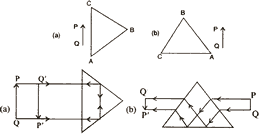Q. 10. Name the reaction which takes place when a slow neutron beam strikes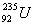nuclei. Write the nuclear reaction involved. Or The work function of lithium is 2.3 eV. What docs it mean? What is the relation between the work function 'W' and threshold wavelengthof a metal? Q. 11. In the series of radioactive disintegration of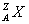first an alpha-particle and then a beta-particle is emitted. What is the atomic number and mass number of the new nucleus formed by these successive disintegrations? Q. 12. In the figure below, circuit symbol of a logic gate and input wave from is shown. (i) Name the logic gate, (ii) write its truth table and (iii) give the output wave form.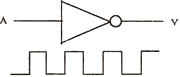Q. 13. What is an equipotential surface? A uniform electric field of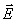300 NC -1 is directed along PQ. A, B and C are three points in the field having x and y coordinates (in metres) as shown in the figure. Calculate potential difference between the points (i) A and B and (ii) B and C.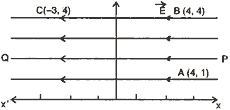Q. 14. Draw a labelled diagram of Van de Graff generator. State its principle of working. Q. 15. What is meant by 'drift velocity of free electrons'? Derive Ohm's law on the basis of the theory of electron drift. Or What is Wheatstone bridge? Deduce the condition for which Wheatstone bridge is balanced. Q. 16. What is meant by the sensitivity of a potentiometer? A battery E1 of 4 V and a variable resistance R h are connected in series with the wire AB of the potentiometer. The length of the wire of the potentiometer is 1 metre. When a cell E 2 of e.m.f. 1.5 volt is connected between points A and C, no current flows through E 2 Length of AC = 60 cm. (i) Find the potential difference between the ends A and B of the potentiometer, (ii) Would the method work, if the battery E